# Green's Theorem

Green's Theorem is a vector identity that is equivalent to the curl theorem in two dimensions. It relates the line integral around a simple closed curve$\partial\Omega$ with the double integral over the plane region$\Omega\,$.

The theorem is named after the British mathematician George Green. It can be applied to various fields in physics, among others flow integrals.

## Mathematical Statement in two dimensions

Let$\Omega\,$ be a region in$\R^2$ with a positively oriented, piecewise smooth, simple closed boundary$\partial\Omega$. f(x,y) and g(x,y) are functions defined on a open region containing$\Omega\,$ and have continuous partial derivatives in that region. Then Green's Theorem states that$\oint\limits_{\partial\Omega}(fdx+gdy)=\iint\limits_\Omega \left(\frac{\partial g}{\partial x}-\frac{\partial f}{\partial y}\right)dxdy$

The theorem is equivalent to the curl theorem in the plane and can be written in a more compact form as$\oint\limits_{\partial \Omega}\mathbf{F}\cdot d\mathbf{S}=\iint\limits_\Omega (\nabla\times\mathbf{F})d\mathbf{A}$

### Application: Area Calculation

Green's theorem is very useful when it comes to calculating the area of a region. If we take f(x,y) = y and g(x,y) = x, the area of the region$\Omega\,$, with boundary$\partial\Omega$ can be calculated by$A=\frac{1}{2}\oint\limits_{\partial \Omega} xdy-ydx$

This formula gives a relationship between the area of a region and the line integral around its boundary.

If the curve is parametrized as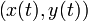$\left(x(t),y(t)\right)$, the area formula becomes$A=\frac{1}{2}\oint\limits_{\partial \Omega}(xy'-x'y)dt$

## Statement in three dimensions

Different ways of formulating Green's theorem in three dimensions may be found. One of the more useful formulations is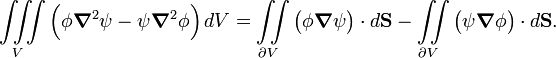$\iiint\limits_V \Big( \phi \boldsymbol{\nabla}^2\psi - \psi \boldsymbol{\nabla}^2\phi\Big)\, d V = \iint\limits_{\partial V} \big(\phi \boldsymbol{\nabla}\psi\big) \cdot d\mathbf{S} - \iint\limits_{\partial V} \big(\psi \boldsymbol{\nabla}\phi\big) \cdot d\mathbf{S}.$

### Proof$\iiint\limits_V \nabla \cdot \mathbf{F} \, d V = \iint\limits_{\partial V}\mathbf{F} \cdot d\mathbf{S}$

where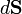$d\mathbf{S}$ is defined by$d\mathbf{S}=\mathbf{n} \, dS$ and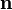$\mathbf{n}$ is the outward-pointing unit normal vector field.

Insert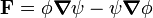$\mathbf{F} = \phi \boldsymbol{\nabla}\psi - \psi \boldsymbol{\nabla}\phi$

and use\begin{align} \boldsymbol{\nabla}\cdot \mathbf{F} &= \big(\boldsymbol{\nabla}\phi\big)\cdot \big(\boldsymbol{\nabla}\psi\big) -\big(\boldsymbol{\nabla}\psi\big)\cdot \big( \boldsymbol{\nabla}\phi\big) + \phi \boldsymbol{\nabla}^2\psi - \psi \boldsymbol{\nabla}^2\phi \\ &= \phi \boldsymbol{\nabla}^2\psi - \psi \boldsymbol{\nabla}^2\phi \end{align}

so that we obtain the result to be proved,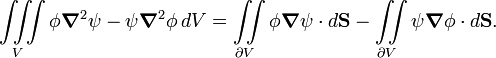$\iiint\limits_V \phi \boldsymbol{\nabla}^2\psi - \psi \boldsymbol{\nabla}^2\phi\, d V = \iint\limits_{\partial V}\phi \boldsymbol{\nabla}\psi \cdot d\mathbf{S} - \iint\limits_{\partial V}\psi \boldsymbol{\nabla}\phi \cdot d\mathbf{S} .$Some content on this page may previously have appeared on Citizendium.